﻿ 木制门套线横截面曲线的数学模型与仿真
 林业科学2013, Vol. 49Issue (11): 141-145PDF
DOI: 10.11707/j.1001-7488.20131120
0

#### 文章信息

Qi Yingjie, Ma Xiaojun, Hu Wanming

Mathematical Model and Simulation of Cross Section Curve for Wooden Door Casing

Scientia Silvae Sinicae, 2013, 49(11): 141-145.
DOI: 10.11707/j.1001-7488.20131120

### 作者相关文章

1. 东北林业大学 哈尔滨 150040;
2. 佳木斯大学 佳木斯 154007

Mathematical Model and Simulation of Cross Section Curve for Wooden Door Casing
Qi Yingjie1, Ma Xiaojun1, 2, Hu Wanming1
1. Northeast Forestry University Harbin 150040;
2. Jiamusi University Jiamusi 154007
Abstract: Wooden door casing is a kind of typical wooden work-pieces with complex-shape curve. In order to solve the problem of machining complex-shape curve by profiling,the mathematical model of cross section curve of the wooden door casing with L-shaped clamping strip was established. The simulation program was writing by using VC++6.0,which could prove the accuracy,versatility and practicability of the mathematical model,which laid the theoretical basis for NC machining of door casing.
Key words: wooden door    door casing    cross section curve    numerical control    mathematical descriptions    analogue simulation

1 门套线数控加工的编程方法

2 门套线横截面曲线数学模型的建立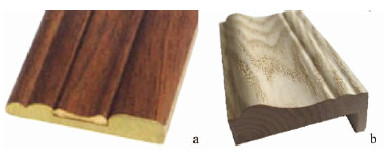图 1 门套线零件 Fig. 1 Work-piece of wooden door casing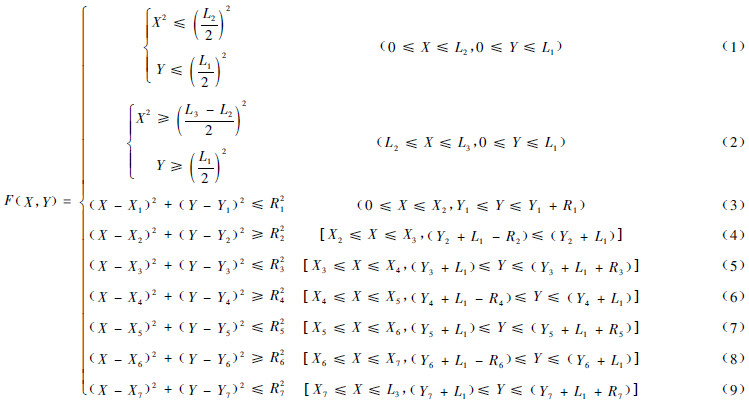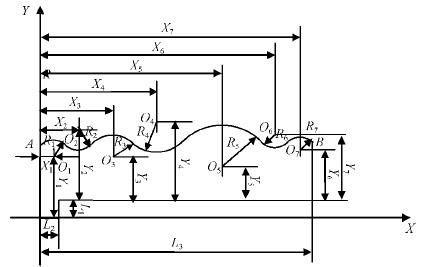图 2 具有L型卡条的门套线横截面曲线的数学模型 Fig. 2 Mathematical model of cross section curve of the wooden door casing with L-shaped clamping strip

 $\begin{array}{l} 2\left( {{X_2} - {X_1}} \right)X + 2\left( {{Y_2} - {Y_1}} \right)Y + X_1^2 + \\ X_2^2 + Y_1^2 + Y_2^2 - R_1^2 + R_2^2 = 0; \end{array}$ (10)
 $\begin{array}{l} 2\left( {{X_3} - {X_2}} \right)X + 2\left( {{Y_3} - {Y_2}} \right)Y + X_2^2 + \\ X_3^2 + Y_2^2 + Y_3^2 - R_2^2 + R_3^2 = 0; \end{array}$ (11)
 $\begin{array}{l} 2\left( {{X_4} - {X_3}} \right)X + 2\left( {{Y_4} - {Y_3}} \right)Y + X_3^2 + \\ X_4^2 + Y_3^2 + Y_4^2 - R_3^2 + R_4^2 = 0; \end{array}$ (12)
 $\begin{array}{l} 2\left( {{X_5} - {X_4}} \right)X + 2\left( {{Y_5} - {Y_4}} \right)Y + X_4^2 + \\ X_5^2 + Y_4^2 + Y_5^2 - R_4^2 + R_5^2 = 0; \end{array}$ (13)
 $\begin{array}{l} 2\left( {{X_6} - {X_5}} \right)X + 2\left( {{Y_6} - {Y_5}} \right)Y + X_5^2 + \\ X_6^2 + Y_5^2 + Y_6^2 - R_5^2 + R_6^2 = 0; \end{array}$ (14)
 $\begin{array}{l} 2\left( {{X_7} - {X_6}} \right)X + 2\left( {{Y_7} - {Y_6}} \right)Y + X_6^2 + \\ X_7^2 + Y_6^2 + Y_7^2 - R_6^2 + R_7^2 = 0。 \end{array}$ (15)

3 门套线横截面曲线的计算机仿真 3.1 门套线横截面曲线实测参数的采集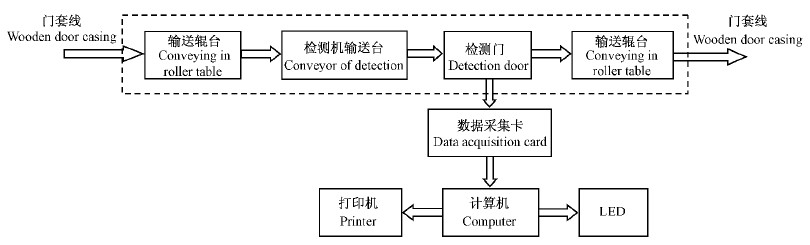图 3 门套线横截面曲线实测参数采集检测系统的工艺流程 Fig. 3 Process flow of actual measurement parameters acquisition and monitoring system of cross section curve of the wooden door casing
3.2 门套线横截面形状的图形仿真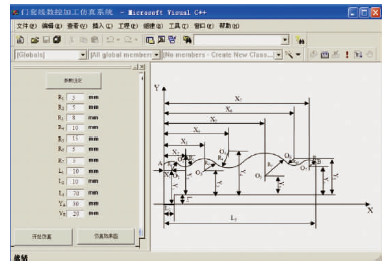图 4 用户界面 Fig. 4 User interface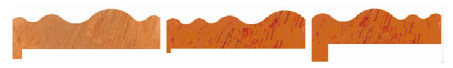图 5 门套线横截面形状仿真效果 Fig. 5 Cross-section effect map of cross section curve of the wooden door casing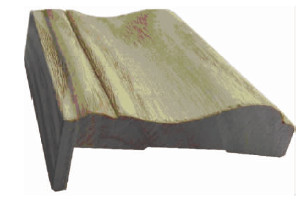图 6 木制门套线的仿真模型 Fig. 6 Physical goods model of wooden door casing
4 结论

1)本文为木制门行业门套线的数控加工提供了数学建模与仿真理论，是将包络加工法与门套线加工工艺结合起来，使传统的工艺方法数学化，为门套线数控加工参数驱动设计以及视频再现提供方法。

2)运用数学分析的基本假设，建立了具有L型卡条的门套线横截面曲线的数学模型，通过对门套线截面曲线描述参数值的仿真结果分析，得到相应描述参数下的门套线横截面形状仿真效果图。结果表明，所建立的数学模型具有良好的精确性、通用性和实用性，可为其他不同类型的门套线截面形状的建模与仿真提供方法。

3)利用门套线横截面曲线的数学模型获得门套线数控加工时，各关键节点坐标变化的参数方程及相应数控加工参数驱动方法可推进木门行业门套线数控加工过程数学描述研究的开展，为门套线数控加工系统的开发奠定理论基础。

  胡海峰,董利红. 2008.规则空间曲面的加工.现代制造, (2/3):114-115.(1)  吕 斌,付跃进,张玉萍. 2008.我国木质门行业的发展现状与趋势. 木材工业,22(5):17-20.(1)  马晓君,马 岩. 2011a.门五金件孔槽数控加工的工业化前景与效益预测. 林产工业,38(2):13-16.(1)  马晓君,马 岩. 2011b.木制门五金件孔槽的数学模型与仿真. 中南林业科技大学学报,31(10):138-144. (1)  马晓君.2012.门五金件安装孔槽数控加工关键技术的研究.哈尔滨:东北林业大学博士学位论文.(1)  马 岩.2006a.中国木材工业数控化的普及. 木材工业,20(2):56-58.(1)  马 岩.2006b.原木形状分段描述方法的数学描述理论研究. 生物数学学报,21 (3): 435-440.(1)  马 岩. 2008.板皮形状与尺寸的数学理论描述. 生物数学学报,23(2):301-305.(2)  马 岩. 2010.板皮形状的计算机仿真与参数驱动理论.计算机应用研究,37(5):45-48.(1)  孟令联,张兆好,徐 杨.2009.现代木门生产工艺与设备. 北京:中国林业出版社.(1)  齐英杰,杨春梅,马 岩.2006.马尾松细胞外廓结构简化模型的建立与仿真. 林业科学,42 (9): 93-95.(1)  齐英杰,夏广岚,马 岩.2010.木制楼梯扶手弯头端截面曲线的数学模型与仿真.林业科学, 49(9):116-119.(1)  齐英杰,马 岩.2008.原木裁板皮过程的建模理论与仿真方法.林业科学,44(12):112-115.(1)  王文波. 2006.数学建模及其基础知识详解. 武汉: 武汉大学出版社,28-62.(1)  吴智慧. 2004.现代木材加工技术第三讲:现代木家具的型面与曲面铣削工艺. 家具,(3):20-25.(1)  徐 杨. 2009.现代实木门的装配工艺及设备. 木工机床,(4):27-29.(1)  徐达丽,马 岩,郭继峰. 2004.直榫榫眼形状的数学描述.东北林业大学学报,32(6):120-121.(1)  张云秀,马 岩,齐英杰. 2004.以数学方法对榫眼形状进行描述. 林业机械与木工设备,32(9):14-16.(1)  朱晓春.2009.数控技术.2版.北京:机械工业出版社,17.(1)  Vinokuro V A,Sadovnichy V A. 2010.Mathematical description of artificial sense of touch systems. Journal of Mathematical Sciences,169(4):413-429.(1)